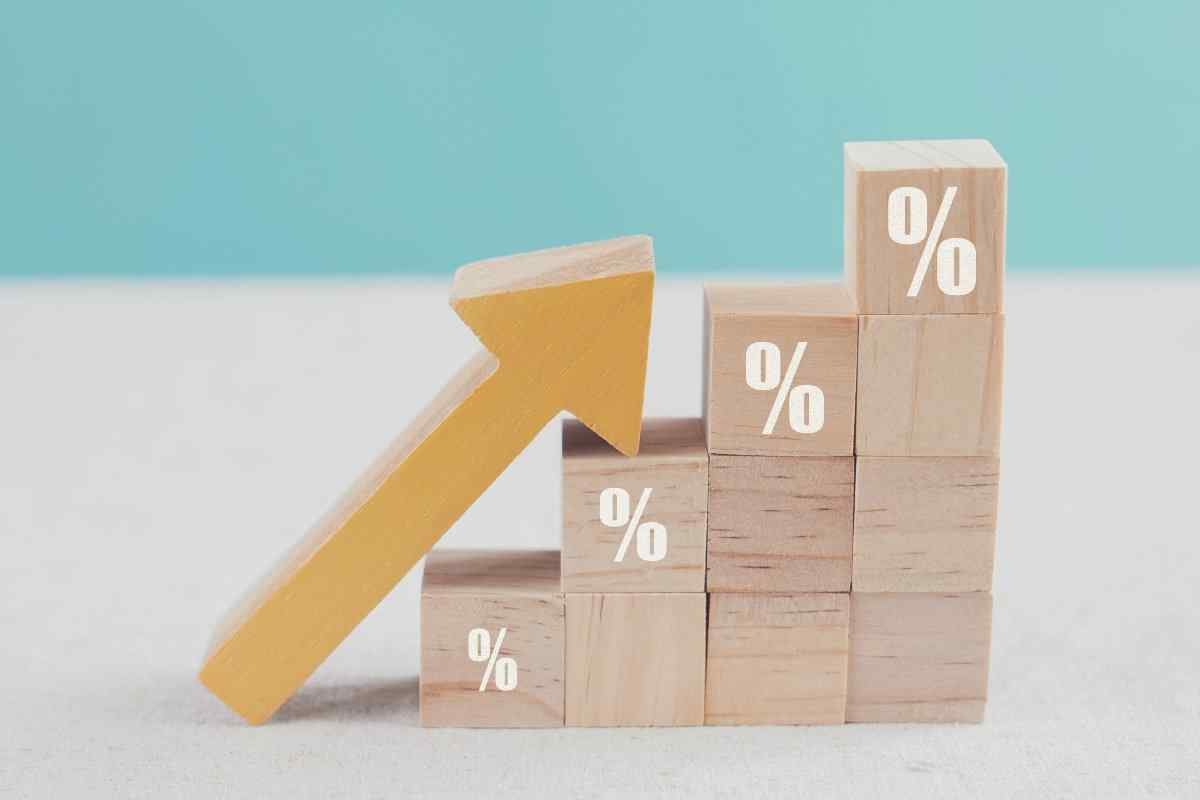# Difference Between Nominal and Real Interest Rate

Date Published: Jul 19, 2022Editor:Reviewer:

In March 2022, the U.S. recorded a 41-year high inflation rate of 8.4%, following 7.0% from February 2021 to February 2022. The 8.4% increase in the Consumer Price Index was the most significant 12-month advance since December 1981. This increase significantly changed the real interest rate of an investment. Therefore, investors should understand the difference between nominal interest rates vs. real interest rates to invest effectively.

## What Is the Nominal Interest Rate?Nominal interest rate refers to the interest rate before inflation is factored in. It remains steady throughout the life of a bond or loan. The rate is calculated by taking into account different factors, such as the demand and supply chains and the inflation rate of the last financial year. Therefore, it doesn’t consider inflation changes during the bond or loan term. Usually, the nominal interest rate is low when there is ample money in circulation.

For example, suppose you have invested \$2,000 in a bond with a 5% per annum interest rate. As a result, you will gain \$100 and have \$2,100 in your account by the end of the year.

## What Is the Real Interest Rate?

Request a Loan Today*
By clicking “Get Started”, I consent and agree to the Privacy Policy and Terms of Site Use.
*By filling out the form above, you will be routed to OpenCashAdvance.com’s loan request form.

While the nominal interest reflects the interest rate gained or paid, the real interest rate is adjusted to remove the effects of inflation, identify the shift in purchasing power, and give the real rate of a bond.

The real interest rate is calculated by subtracting the nominal interest rate from the inflation rate.

Let’s follow the above example and suppose you have invested \$2,000 in a bond. You are offered a 5% per annum interest rate, which means you will have \$2,100 by the end of the term. However, suppose that the inflation rate by the end of the year is 4%. As a result, you would only be earning 1% real interest.

This is because the prices of goods rise, but your nominal interest does not. Therefore, your buying power diminishes because you need more money to buy the same amount of goods, which means that your \$100 profit ceases to have the same purchasing power. Although you have still earned 5% nominal interest, in reality, the value of the money has diminished because of higher inflation rates. As such, the real interest rate reflects your money’s purchasing power in relation to inflation.

## What Is the Difference Between Nominal and Real Interest Rates?

Nominal and real interest rates reveal the sum a person will earn on an investment. Nonetheless, they have significant differences. The main difference is that the nominal interest remains stable over the course of the investment period. Conversely, the real interest rate fluctuates based on the inflation rate.

Moreover, inflation can reduce the value of your money. Therefore, calculating the return rate in real value rather than in nominal offers a clearer picture of the investment's success and the actual return after adjusting for inflation. Consequently, investors can determine whether they should take more risks or exit the market.

Below is a comparison table between the nominal rate and the real rate:

 Parameters of Comparison Nominal Interest Rate Real Interest Rate Definition The market rate charged or earned The effective rate that the investor will earn Inflation It is calculated by considering the inflation rate of the last financial year and doesn't take current inflation into account It is calculated by considering the difference between the nominal interest rate and the current inflation rate Adjustment and Stability It is stable and doesn't require adjustments It is adjusted to the current inflation rate Measure It cannot be negative It can be negative if the inflation rate exceeds the nominal interest rate

## Sum up

The nominal interest rate is a tool to determine the return on investment.  The nominal interest rate is the percentage of return on investment, whereas the real interest rate considers inflation to measure the actual value of an investment. Therefore, investors should keep an eye on both nominal and real interest rates because the nominal rate will reveal the state of the market, whereas the real rate will disclose the actual returns from the market.